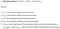# Why is diversification a no-brainer?

We all (hopefully) know that smart diversification is an essential part of a good investment plan. However, when we see assets skyrocketing, it is tempting to want to put all you eggs in one basket.

Before we continue, bear in mind that I will be using the terms volatility, risk and standard deviation interchangeably. Note that in portfolio analysis, they mean the same thing.

• Nukia’s expected return is a bit lower, at 15%. However, the risk is also lower, at 7%.
`### characteristics of each assetgame_step = {'expected_return': 0.25, 'volatility': 0.15}nukia = {'expected_return': 0.15, 'volatility': 0.07}correlation = 0.3`

## Sharpe Ratio

Well, if you had to choose just one, a good way to decide is to look at Sharpe Ratio of each one. It is a benchmark measure of the most important trade-off in investments: risk vs return

## Portfolio Return

Let’s start off with the combined returns. It is very easy to calculate, since it will be the weighted average of the returns of GameStep and Nukia.

`def calculate_portfolio_returns(weight_a, weight_b):    ret_a = weight_a * game_step['expected_return']    ret_b = weight_b * nukia['expected_return']    combined_return = ret_a + ret_b    return combined_return`

## Portfolio Risk

On the other hand, the portfolio volatility is not a simple weighted average. If you diversify, it will be lower than the simple weighed average as long as both assets are not perfectly correlated.

## Let’s get technical

If you want to go deeper into it, here is the formula for the portfolio variance (feel free to skip to the next session if you prefer):You don`t need to memorize it. The takeaway is that portfolio volatility is not a linear combination of the assets volatility.
`# creates list of 21 diferent ratio combinations allocation_strategies = [[w, 1-w] for w in np.linspace(0, 1, 21)]# calculates the portfolio volatility correlation = 0.3def calculate_portfolio_vol(weight_a, weight_b, correlation):    vol_a = game_step['volatility']    vol_b = nukia['volatility']    portf_variance_formula =  (           ( weight_a**2 ) * (vol_a**2)        + ( weight_b**2 ) * (vol_b**2 )        + 2*correlation*weight_a*weight_b*vol_a*vol_b                              )                                  portf_volatility = portf_variance_formula**(1/2)    return portf_volatility`
`# creates list of different allocation strategiesallocation_strategies = [[w, 1-w] for w in np.linspace(0, 1, 21)]# calculates the portfolio return and volatility # for each allocation strategyimport pandas as pddef test_allocation(allocation_strategies, correlation):    d = {}    portfolio_returns = []    portfolio_volatility = []    for allocation in allocation_strategies:        weight_a = allocation        weight_b = allocation                        combined_return = calculate_portfolio_returns(            weight_a,             weight_b)                portfolio_returns.append(combined_return)                combined_vol = calculate_portfolio_vol(            weight_a,             weight_b,             correlation)                portfolio_volatility.append(combined_vol)        d['returns'] = portfolio_returns    d['volatility'] = portfolio_volatility    return pd.DataFrame(d)portfolios_df = test_allocation(allocation_strategies, correlation)## plots the figures# creates figure and subplotsfig, axs = plt.subplots(10, 10,                         figsize=(10, 10),                         sharex=True)ax1 = plt.subplot2grid(shape=(10, 7),                        loc=(0,0),                        colspan=10,                        rowspan=7)ax3 = plt.subplot2grid(shape=(10, 3),                        loc=(7,0),                        colspan=10,                        rowspan=3)### plots the portfolio returns curvecolor = 'tab:blue'ax1.set_ylabel('Returns', color = color)  ax1.plot(alloc_labels,          portfolios_df['returns'],          color = color)  ax1.tick_params(axis ='y',                 labelcolor = color)ax1.tick_params(axis ='x',                 labelcolor = 'white')  # Adding Twin Axes to plot portfolio volatility on the same subplot as ax1 ax2 = ax1.twinx()### plots the portfolio returns curvecolor = 'tab:green'ax2.set_ylabel('Volatility',                color = color)  ax2.plot(alloc_labels,          portfolios_df['volatility'], color = color)  ax2.tick_params(axis ='y',                 labelcolor = color)# Adding title plt.title('Risk/Return for different allocation strategies',           fontweight ="bold")ax3.plot(alloc_labels,             portfolios_df['sharpe'],             color='gray',           label='Sharpe Ratio')ax3.set_ylabel('Sharpe Ratio', color = 'black') ax3.tick_params(axis ='y', labelcolor = 'black')ax3.tick_params(axis ='x', labelcolor = 'gray', rotation=45,)ax3.set_xlabel('allocation strategies')  plt.show()`

## Visualizing the Portfolio Curve

To get a better picture of how the portfolio performs for each allocation strategy, consider the chart below, that shows volatility (x-axis) and returns (y-axis):

`# portfolio curve chartplt.figure(figsize=(12, 7))plt.plot(portfolios_df['volatility'],         portfolios_df['returns'], color='purple'        )plt.title('Portfolio Curve', fontsize=20)plt.xlabel('Volatility')plt.ylabel('Return')plt.scatter(nukia['volatility'], nukia['expected_return'], color='red')plt.scatter(nukia['volatility'], 0.1665            , color='blue')plt.show()# portfolio curves for different correlationsplt.figure(figsize=(12, 7))for corr in np.linspace(0.1, 1, 10):    corr_df = test_allocation(allocation_strategies, corr)        plt.plot(corr_df['volatility'],         corr_df['returns'], label=round(corr, 1)        )plt.title('Portfolio Curves for Different Correlation Levels', fontsize=20)plt.xlabel('volatility')plt.ylabel('return')plt.legend()plt.show()# sharpe ratios for different correlationsplt.figure(figsize=(12, 7))for corr in np.linspace(0.1, 1, 10):    corr_df = test_allocation(allocation_strategies, corr)        plt.plot(alloc_labels,         corr_df['sharpe'], label=round(corr, 1)        )plt.title('Sharpe Ratio for Different Correlation Levels', fontsize=20)plt.tick_params(axis='x', rotation=45)plt.xlabel('allocation')plt.ylabel('Sharpe Ratio')plt.legend()plt.show()`